# Unit 3 Boolean Expressions if Statements Compound Boolean

• Slides: 30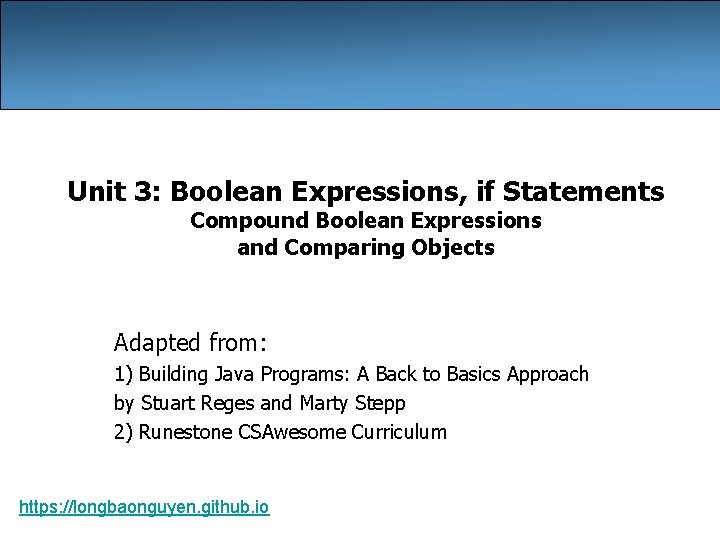Unit 3: Boolean Expressions, if Statements Compound Boolean Expressions and Comparing Objects Adapted from: 1) Building Java Programs: A Back to Basics Approach by Stuart Reges and Marty Stepp 2) Runestone CSAwesome Curriculum https: //longbaonguyen. github. ioEvaluating logic expressions Sometimes it is useful to use nested if conditions: if statements within if statements. // if x is odd if(x % 2 != 0){ // if x is positive if(x > 0){ … } } We can combine the above nested if conditions using logical operators. 2Logical operators • Tests can be combined using logical operators: Operator Description Example Result && and (2 == 3) && (-1 < 5) false || or (2 == 3) || (-1 < 5) true ! not !(2 == 3) true • "Truth tables" for each, used with logical values p and q: p q p && q p || q true true false true false p true !p false true 3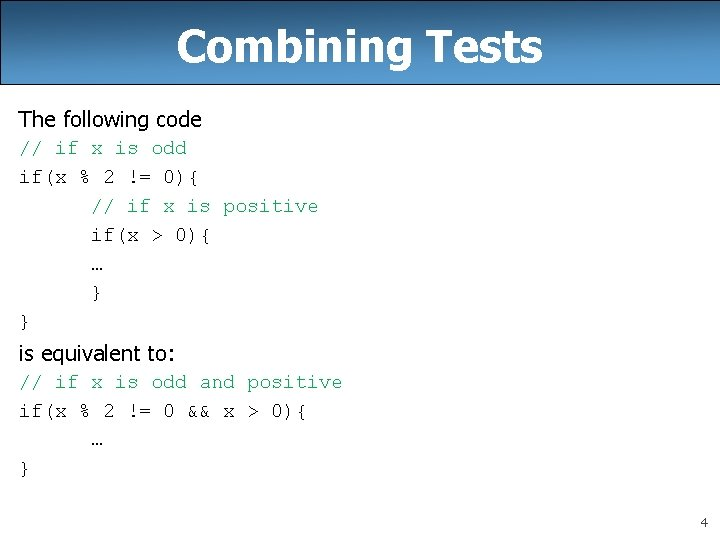Combining Tests The following code // if x is odd if(x % 2 != 0){ // if x is positive if(x > 0){ … } } is equivalent to: // if x is odd and positive if(x % 2 != 0 && x > 0){ … } 4Using boolean good. Age = age >= 21 && age < 29; boolean good. Height = height >= 78 && height < 84; boolean rich = salary >= 100000. 0; if ((good. Age && good. Height) || rich) { System. out. println("Okay, let's go out!"); } else{ System. out. println("It's not you, it's me. . . "); } 5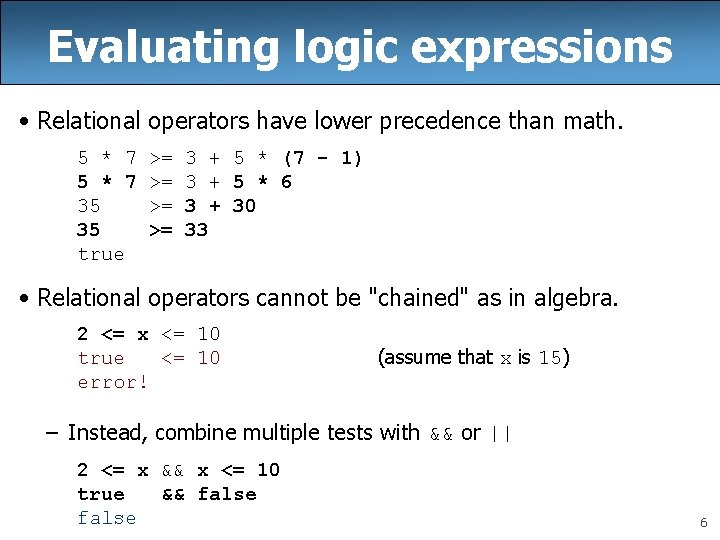Evaluating logic expressions • Relational operators have lower precedence than math. 5 * 7 35 35 true >= >= 3 + 5 * (7 - 1) 3 + 5 * 6 3 + 30 33 • Relational operators cannot be "chained" as in algebra. 2 <= x <= 10 true <= 10 error! (assume that x is 15) – Instead, combine multiple tests with && or || 2 <= x && x <= 10 true && false 6Order of Operations Precedence Operator Operation highest ** exponentiation - negation *, /, % multiplication, division, modulo +, - adding, subtraction ==, !=, <, >, <=, >= comparisons(relationals) not logical not and logical and or logical or = assignment lowest 7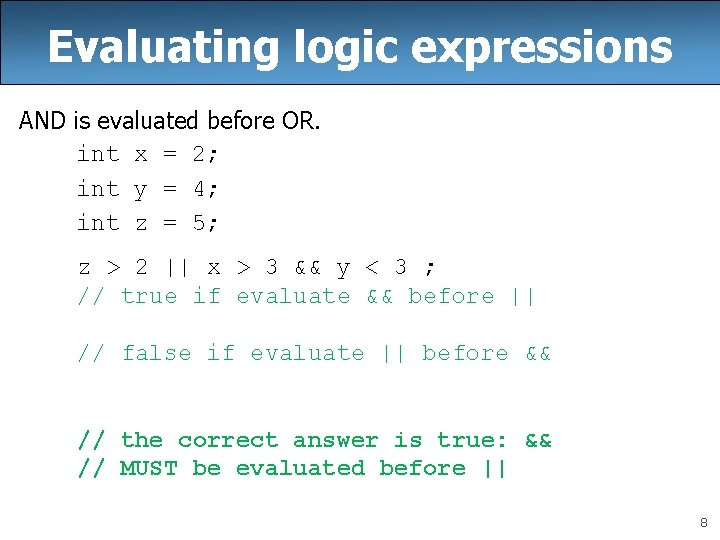Evaluating logic expressions AND is evaluated before OR. int x = 2; int y = 4; int z = 5; z > 2 || x > 3 && y < 3 ; // true if evaluate && before || // false if evaluate || before && // the correct answer is true: && // MUST be evaluated before || 8Logical questions • What is the result of each of the following expressions? int x = 42; int y = 17; int z = 25; – – – y < x && y <= z x % 2 == y % 2 || x % 2 == z % 2 x <= y + z && x >= y + z !(x < y && x < z) (x + y) % 2 == 0 || !((z - y) % 2 == 0) Answers: - true - false 9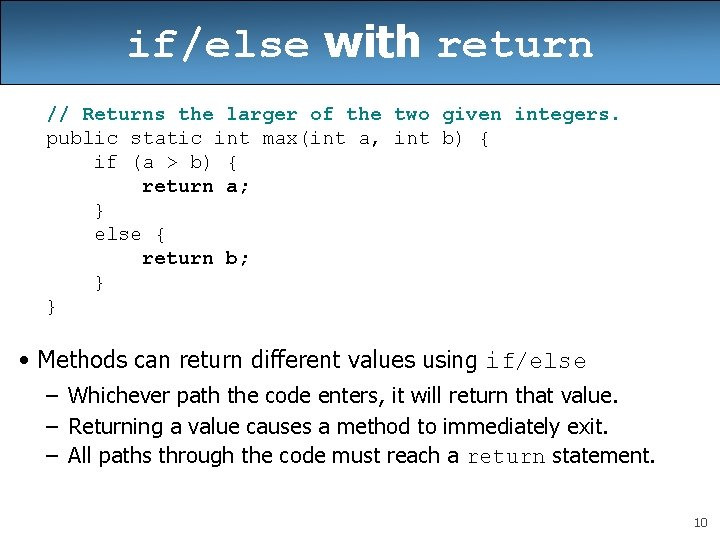if/else with return // Returns the larger of the two given integers. public static int max(int a, int b) { if (a > b) { return a; } else { return b; } } • Methods can return different values using if/else – Whichever path the code enters, it will return that value. – Returning a value causes a method to immediately exit. – All paths through the code must reach a return statement. 10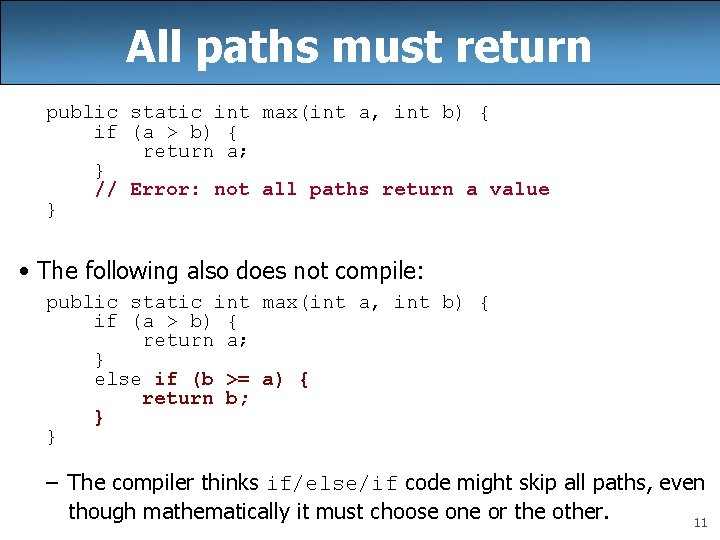All paths must return public static int max(int a, int b) { if (a > b) { return a; } // Error: not all paths return a value } • The following also does not compile: public static int max(int a, int b) { if (a > b) { return a; } else if (b >= a) { return b; } } – The compiler thinks if/else/if code might skip all paths, even though mathematically it must choose one or the other. 11Correction public static int max(int a, int b) { if (a > b) { return a; } else{ return b; } } OR public static int max(int a, int b) { if (a > b) { return a; } return b; } 12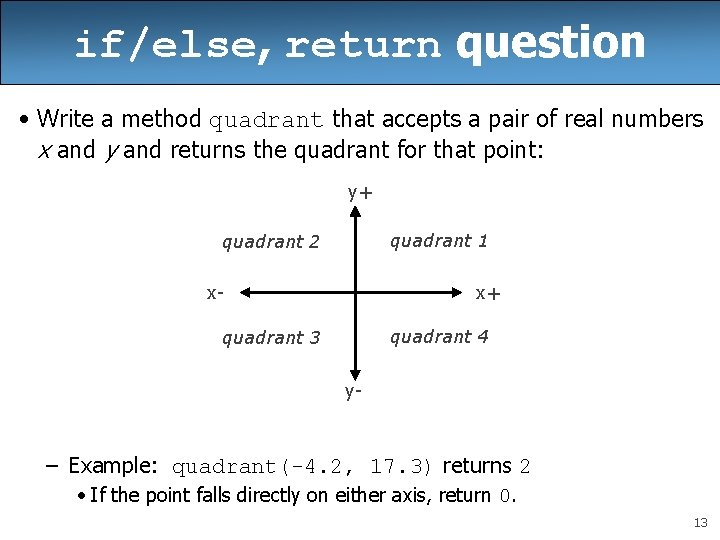if/else, return question • Write a method quadrant that accepts a pair of real numbers x and y and returns the quadrant for that point: y+ quadrant 1 quadrant 2 x- x+ quadrant 4 quadrant 3 y- – Example: quadrant(-4. 2, 17. 3) returns 2 • If the point falls directly on either axis, return 0. 13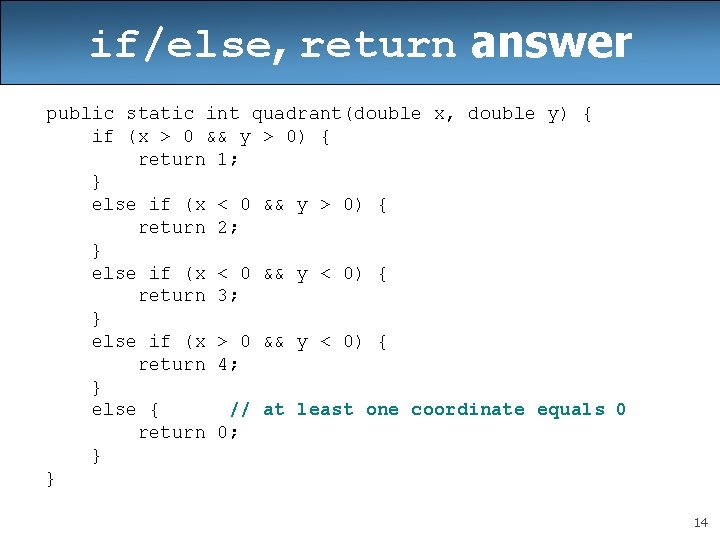if/else, return answer public static int quadrant(double x, double y) { if (x > 0 && y > 0) { return 1; } else if (x < 0 && y > 0) { return 2; } else if (x < 0 && y < 0) { return 3; } else if (x > 0 && y < 0) { return 4; } else { // at least one coordinate equals 0 return 0; } } 14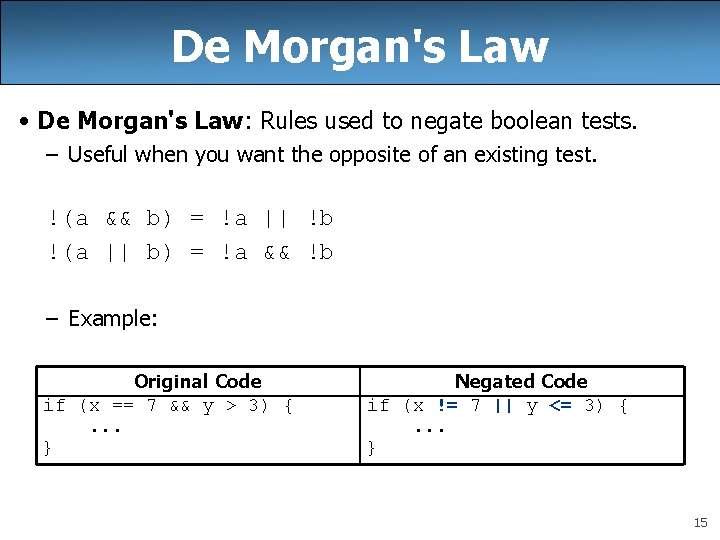De Morgan's Law • De Morgan's Law: Rules used to negate boolean tests. – Useful when you want the opposite of an existing test. !(a && b) = !a || !b !(a || b) = !a && !b – Example: Original Code if (x == 7 && y > 3) {. . . } Negated Code if (x != 7 || y <= 3) {. . . } 15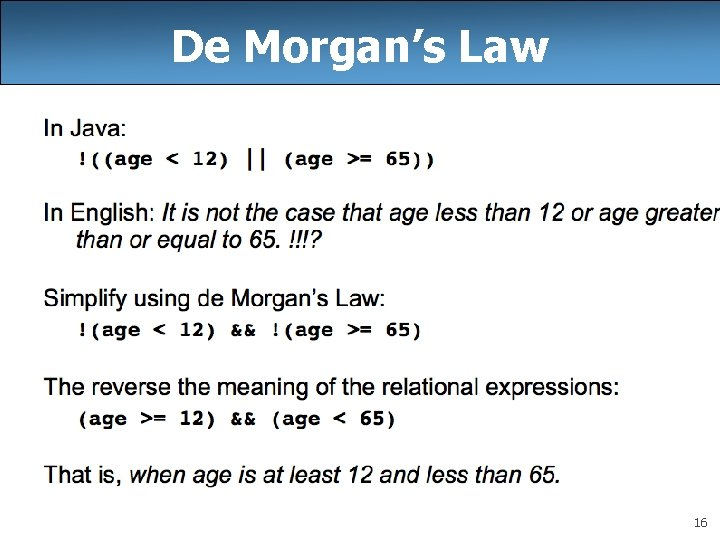De Morgan’s Law 16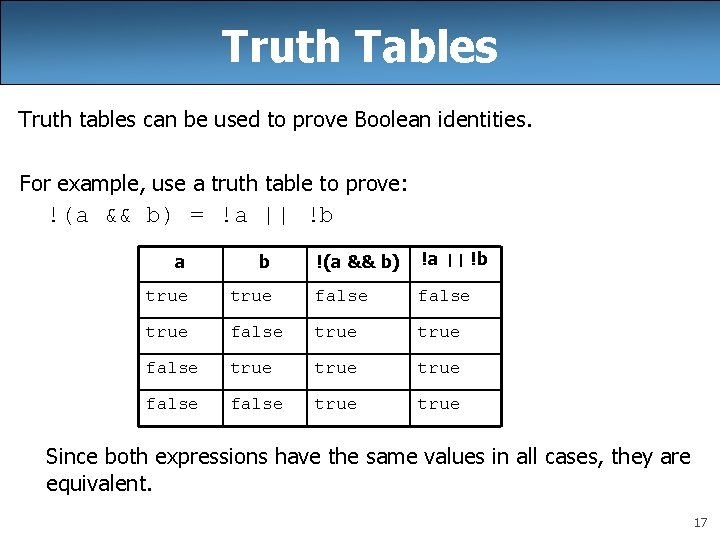Truth Tables Truth tables can be used to prove Boolean identities. For example, use a truth table to prove: !(a && b) = !a || !b !(a && b) !a || !b true false true false true a b true Since both expressions have the same values in all cases, they are equivalent. 17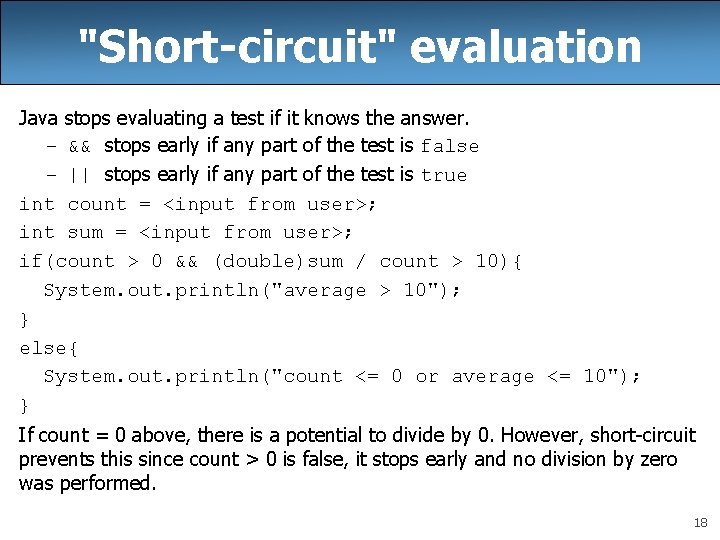"Short-circuit" evaluation Java stops evaluating a test if it knows the answer. – && stops early if any part of the test is false – || stops early if any part of the test is true int count = <input from user>; int sum = <input from user>; if(count > 0 && (double)sum / count > 10){ System. out. println("average > 10"); } else{ System. out. println("count <= 0 or average <= 10"); } If count = 0 above, there is a potential to divide by 0. However, short-circuit prevents this since count > 0 is false, it stops early and no division by zero was performed. 18Boolean practice questions Write a method named is. Vowel that returns whether a String is a vowel (a, e, i, o, or u). Assume all letters are lowercase. – is. Vowel("q") returns false – is. Vowel("a") returns true – is. Vowel("e") returns true public static boolean is. Vowel(String s) { return s. equals("a") || s. equals("e") || s. equals("i") || s. equals("o") || s. equals("u"); } 19Boolean practice questions Change the above method into an is. Non. Vowel method that returns whether a String is any character except a vowel. – is. Non. Vowel("q") returns true – is. Non. Vowel("a") returns false – is. Non. Vowel("e") returns false What’s the wrong strategy? // Enlightened "Boolean Zen" version public static boolean is. Non. Vowel(String s) { return !s. equals("a") && !s. equals("e") && !s. equals("i") && !s. equals("o") && !s. equals("u"); } 20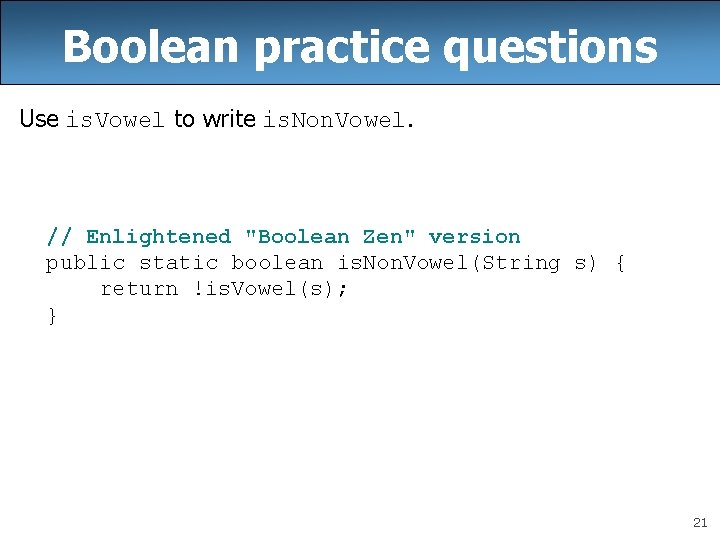Boolean practice questions Use is. Vowel to write is. Non. Vowel. // Enlightened "Boolean Zen" version public static boolean is. Non. Vowel(String s) { return !is. Vowel(s); } 21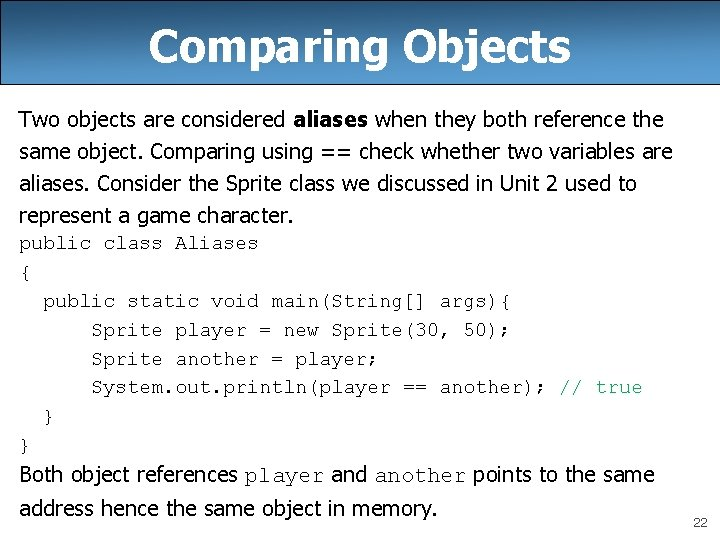Comparing Objects Two objects are considered aliases when they both reference the same object. Comparing using == check whether two variables are aliases. Consider the Sprite class we discussed in Unit 2 used to represent a game character. public class Aliases { public static void main(String[] args){ Sprite player = new Sprite(30, 50); Sprite another = player; System. out. println(player == another); // true } } Both object references player and another points to the same address hence the same object in memory. 22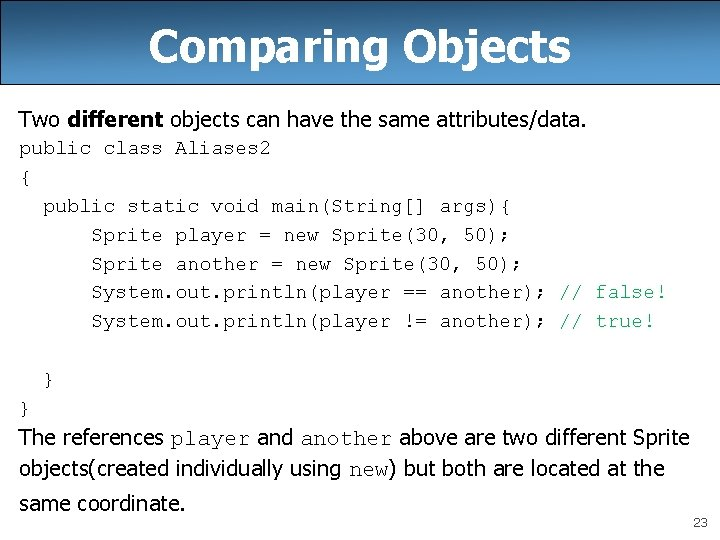Comparing Objects Two different objects can have the same attributes/data. public class Aliases 2 { public static void main(String[] args){ Sprite player = new Sprite(30, 50); Sprite another = new Sprite(30, 50); System. out. println(player == another); // false! System. out. println(player != another); // true! } } The references player and another above are two different Sprite objects(created individually using new) but both are located at the same coordinate. 23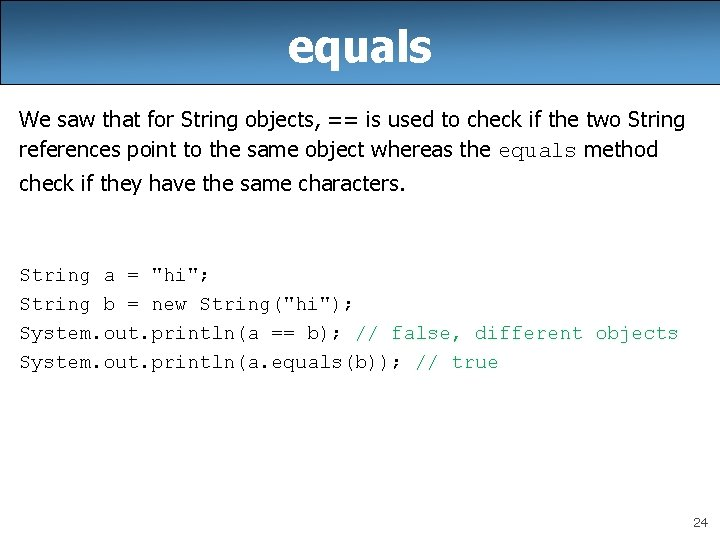equals We saw that for String objects, == is used to check if the two String references point to the same object whereas the equals method check if they have the same characters. String a = "hi"; String b = new String("hi"); System. out. println(a == b); // false, different objects System. out. println(a. equals(b)); // true 24equals Later in Unit 5 when we write our own objects, it will be useful to implement the equals method for our class so check whether two different objects are equivalent(same data). For example, consider Point objects with attributes x and y representing points on the plane. Although the following two points are distinct programmatically. They are equivalent mathematically. The equals method will allow us to detect this. More on this later. Point a = new Point(3, 4); Point b = new Point(3, 4); System. out. println(a == b); // false, different objects System. out. println(a. equals(b)); // true 25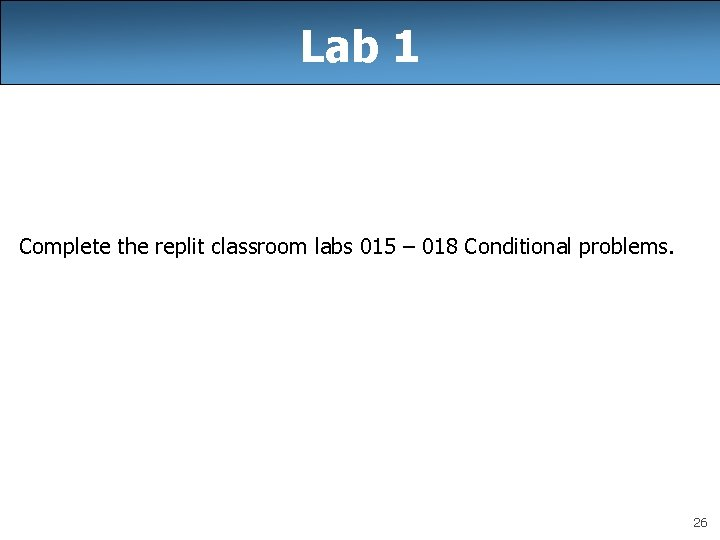Lab 1 Complete the replit classroom labs 015 – 018 Conditional problems. 26Lab 2: BMI Create a new repl for this lab. BMI Formula for body mass index (BMI): Weight class below 18. 5 underweight [18. 5 – 25) normal [25. 0 – 30) overweight 30. 0 and up obese Write a program that produces output like the following. Use Scanner for input. Height (in inches): 70. 0 Weight (in pounds) 194. 25 BMI = 27. 868928571428572 Overweight 27Lab 2: BMI Your program must include two methods: 1) the method bmi which takes two double parameters height and weight and returns the bmi and 2) the method weight. Class which takes two double parameters height and weight and returns a string classifying the weight class. The weight. Class method must call the bmi method! public static double bmi(double height, double weight) {…} public static String weight. Class(double height, double weight) {…} 28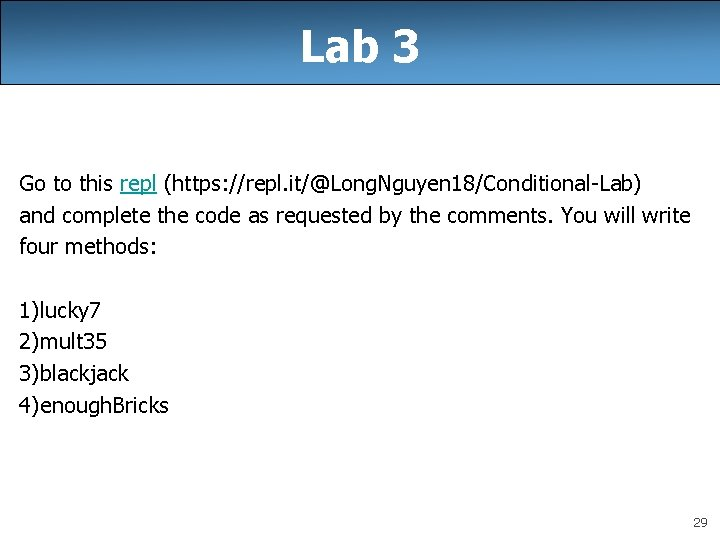Lab 3 Go to this repl (https: //repl. it/@Long. Nguyen 18/Conditional-Lab) and complete the code as requested by the comments. You will write four methods: 1)lucky 7 2)mult 35 3)blackjack 4)enough. Bricks 29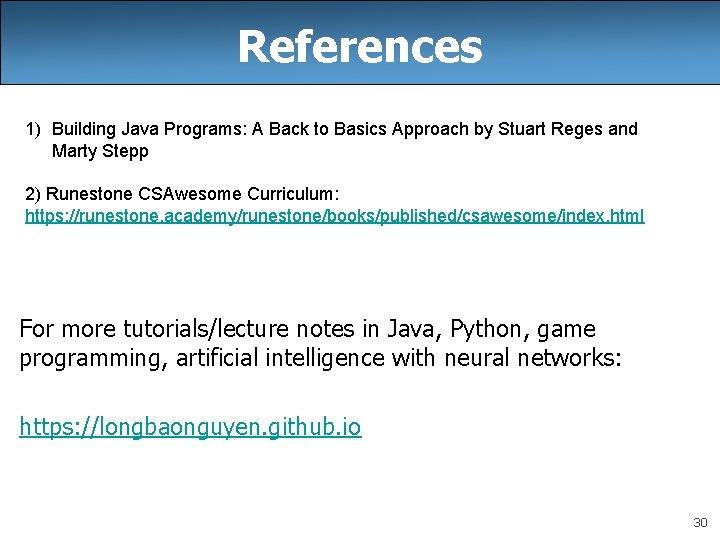References 1) Building Java Programs: A Back to Basics Approach by Stuart Reges and Marty Stepp 2) Runestone CSAwesome Curriculum: https: //runestone. academy/runestone/books/published/csawesome/index. html For more tutorials/lecture notes in Java, Python, game programming, artificial intelligence with neural networks: https: //longbaonguyen. github. io 30